# Signal decimation using a compensated CIC filter

2016-10-03

Decimation is the process of converting a time-domain signal sampled at frequency $f_s$ into a signal sampled at frequency $f_s / R$, where $R$ is the decimation rate. Downsampling alone, i.e. keeping one sample every $R$ samples is not sufficient. A low-pass filtering step must precede downsampling to prevent aliasing.

## Low-pass filtering using a FIR filter

The ideal low-pass filter has a unity frequency response between $0$ and $f_s/(2R)$ and a null response between $f_s/(2R)$ and $f_s/2$. In the time-domain, this filter is equivalent to a convolution with a sinc function. In pratice, the convolution must be performed with a finite number of coefficients. Such filters are called Finite Impulse Response filters (FIR). The greater the number of coefficients, the better the filter will be able to approximate the ideal response.

Let's say we want to find a FIR filter that approximates an ideal low-pass filter for a decimation rate $R = 4$. The figure below shows the frequency response of the obtained FIR filter for 16, 64 and 256 coefficients.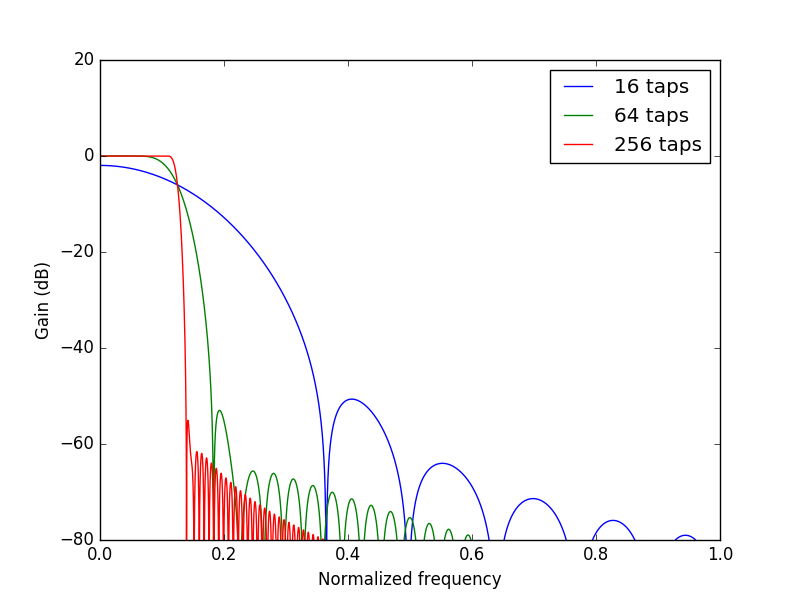A simple implementation of the 256-tap FIR filter would require 256 multiplications and 255 additions at each clock cycle. Taking advantage of the coefficient symmetry, one could divide by two the number of multiplications. The number of multiplications can however quickly become a problem for hardware implementation, especially for large decimation rates where the number of coefficients must increase to keep a sharp cutoff.

## From the moving average to the CIC filter

The moving average filter is a simple FIR filter in which all the coefficients are equal. A decimation of rate 4 could be achieved using 4 coefficients equal to one, thus using only 3 additions and 0 multiplications at each clock cycle:

$$y[n] = \sum_{k=0}^{R-1} x[n-k].$$

This is very interesting because multiplications consume much more hardware resources than additions. Note that the frequency response of the moving average filter is a sinc function (Fourier transform of the box car function) which is far from ideal. We will show in the following that this frequency response can be compensated by a well chosen FIR filter . Before going any further, we can note that the moving average formula can be written in recursive form:

$$y[n] = y[n-1] + x[n] - x[n-R].$$

This identity is interesting for large decimation rates since it only requires to compute one addition and one subtraction instead of $R - 1$ additions. This idea is the basis of cascaded-integrator combs (CIC), a class of filters that perform an efficient implementation of moving-average filters.

## Hardware implementation

Let's assume we want to decimate the Red Pitaya ADC stream (125 Msps) by a factor 512 to obtain a 244 kSps stream of decimated ADC data. We will use a CIC filter of rate $R = 256$ followed by a half-band FIR filter that will compensate the frequency response of the CIC filter and decimate by an additional factor 2. The frequency response of a CIC filter is given by :

$$H(f) = \left[ \frac{\sin(\pi RMf)}{\sin(\pi f)} \right]^N,$$

where $N$ is the number of CIC stages, $R$ is the decimation rate, and $M$ is the differential delay. The CIC compiler provided by Xilinx Vivado® allows us to choose the number of stages between 3 and 6, the decimation rate between 4 and 8192, and the differential delay between 1 and 2. We use the following parameters: $N=6$, $R=256$ and $M=1$. Using such a large number of stages increases the out-of-band rejection at the cost of an increased pass-band droop.

The figure below shows the effect of a well chosen 128-taps half-band FIR filter that compensates for the CIC filter frequency response. Note that the FIR filter need only to process data with a rate of 125 Msps / 256 = 488 ksps. The FIR core provided by Xilinx automatically pipelines its architecture in order to minimize the number of multipliers that need to run simultaneously. Multipliers are implemented using dedicated logic elements called DSP slices.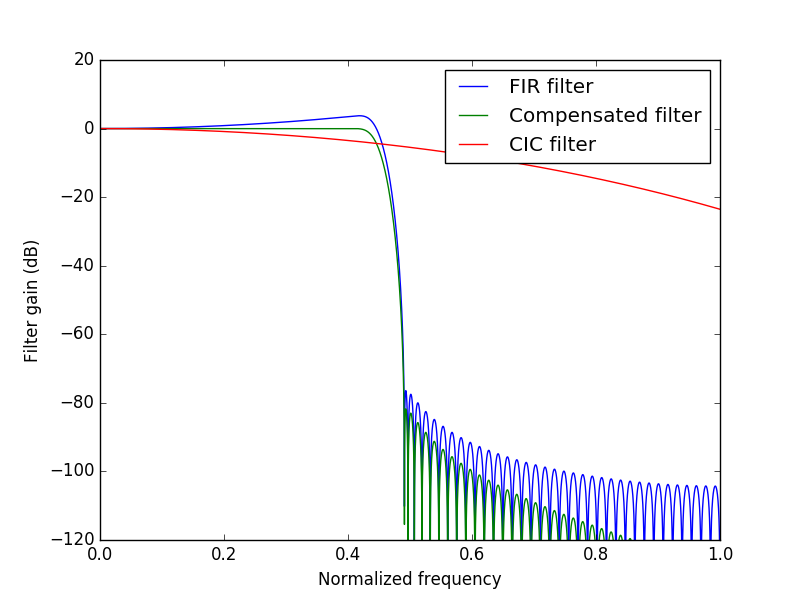The Vivado block design is shown below. The ADC data and its corresponding 125 MHz differential clock enter the FPGA (1) and is converted in a 125 MHz signal adc_dac/adc1[13:0], synchronous with a phase-locked 125 MHz clock adc_dac/adc_clk (2). The rest of the data path uses standard Xilinx blocks connected using AXI4 Stream interface and running at 200 MHz. A clock converter (3) converts the ADC signal from the 125 MHz ADC clock to the 200 MHz interconnect clock FCLK0. Data then goes through the CIC core (4) and the FIR core (5) to enter the AXI-Stream FIFO (6). The AXI-Stream FIFO (16384 x 32 bits) is connected via an AXI4-Lite interface to the M_AXI_GP0 port of the processing system (8) via the interconnect (7). The FIFO can thus be read from Linux as a memory-mapped region. The FIFO is filled at a rate of 244 kSps, which is sufficiently slow to record a continuous data stream without losing any samples.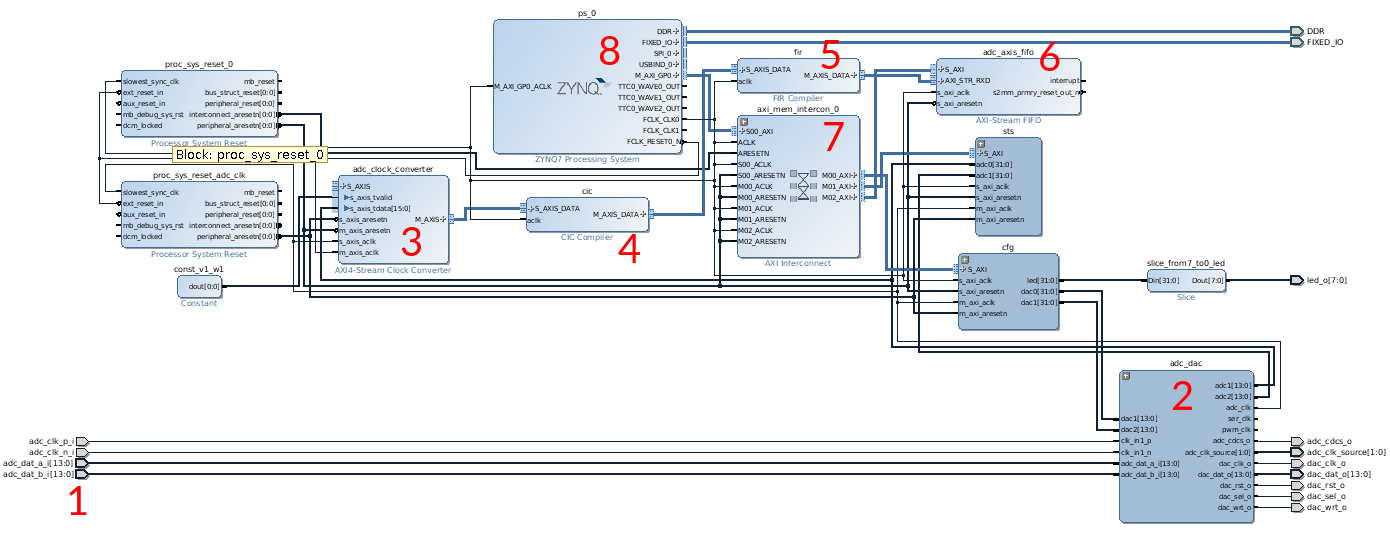## Characterization of the frequency response

We used an Agilent 33220A arbitrary waveform generator to characterize the frequency response of the compensated CIC filter we implemented on the Red Pitaya. Control of both instruments was performed with Python using Ethernet connections.

The transfer function was obtained by sending sine waveforms of 0.5 Vpp amplitude with 4096 frequencies evenly spaced between 0 and the Nyquist frequency (122.07 kHz). These frequencies were chosen to coincide with the frequency bins of the 8192 samples read each time from the Red Pitaya FIFO. The measured transfer function is shown in the graph below, along with the expected theoretical response (the gains were normalized by their maximum for easy comparison):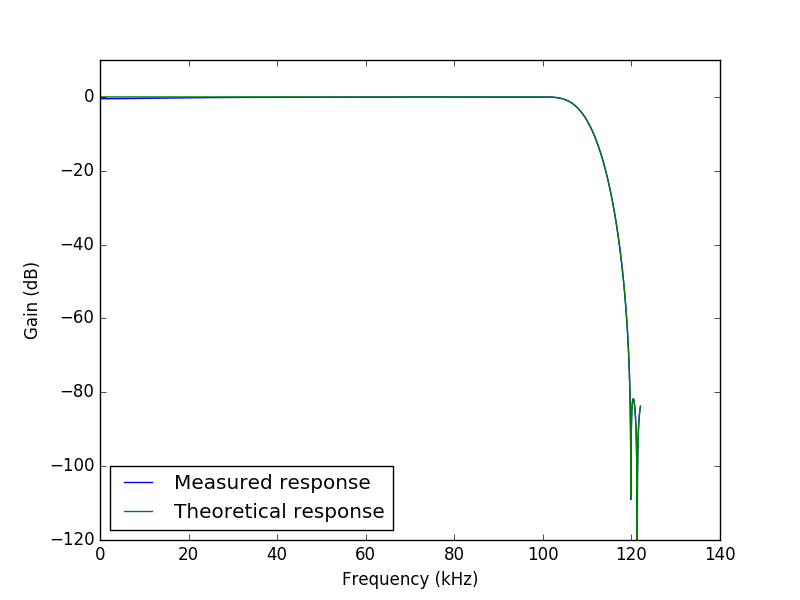The measurement seems to be in very good agreement with the theory. However, a more careful look at the pass-band zone shows that the measured gain is almost 0.5 dB smaller than expected at low frequencies. This is caused by the analog input stage of the Red Pitaya .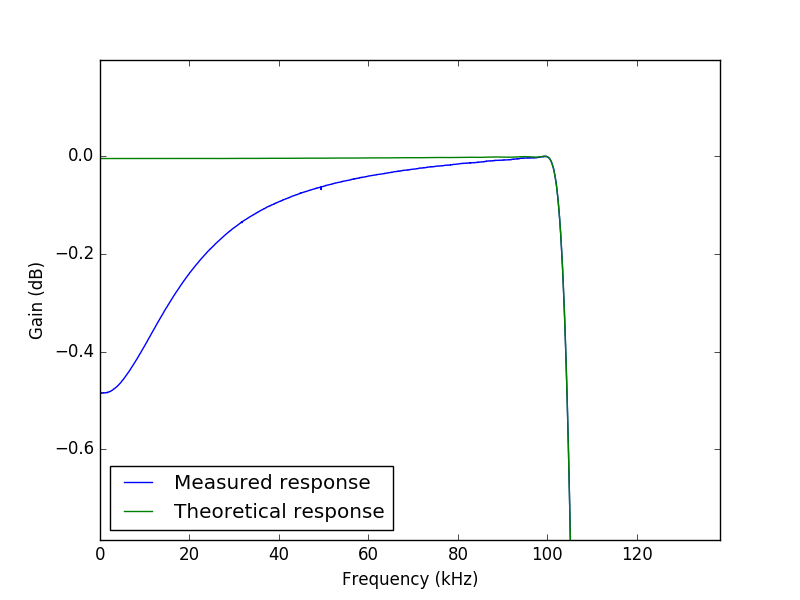## Final thoughts

We did not discuss the effects of coefficient and data quantization in the FIR filter. For simplicity, we used 32-bit width for both data and coefficients. As a result, the FIR filter uses 7 DSP slices. With widths of 24 bits for input data and 18 bits for the coefficient, it would be possible to use only 1 DSP slice! An interesting discussion of the effects of quantization can be found on Andrew Casper's blog Quantized.

One can also note that the shape of the CIC filter is independent of the decimation rate. That means that a fixed FIR filter can compensate a CIC filter with variable decimation rate. You can find on Pavel Demin's website a nice example of a Red Pitaya-based SDR transceiver that uses this property.

## Source code

https://github.com/Koheron/koheron-sdk/tree/master/examples/red-pitaya/decimator